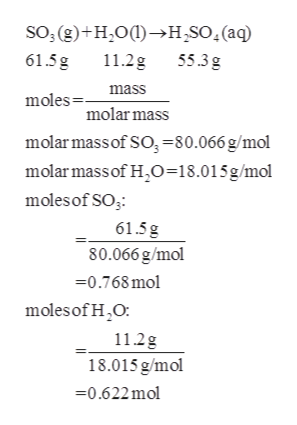# Consider the following reaction between sulfur trioxide and water: SO3(g)+H2O(l)→H2SO4(aq) A chemist allows 61.5 g of SO3 and 11.2 g of H2O to react. When the reaction is finished, the chemist collects 55.3 g of H2SO4 .Determine the limiting reactant for the reaction.Express your answer as a chemical formula.

Question

Consider the following reaction between sulfur trioxide and water:
SO3(g)+H2O(l)→H2SO4(aq) A chemist allows 61.5 g of SO3 and 11.2 g of H2O to react. When the reaction is finished, the chemist collects 55.3 g of H2SO4 .

Determine the limiting reactant for the reaction.

check_circleExpert Solution
Step 1

From the given mass of sulfur trioxide and water...help_outlineImage Transcriptioncloseso, (g)+H20()H,SO.(aq) 61.5g 11.2g 55.3g mass moles molar mass molarmassof SO,-80.066 g/mol molar massof H2O=18.015 g/mol moles of SO 61.5g 80.066 g/mol 0.768mol moles of H2O: 11.2g 18.015 g/mol 0.622mol fullscreen

### Want to see the full answer?

See Solution

#### Want to see this answer and more?

Solutions are written by subject experts who are available 24/7. Questions are typically answered within 1 hour*

See Solution
*Response times may vary by subject and question
Tagged in

### Physical Chemistry